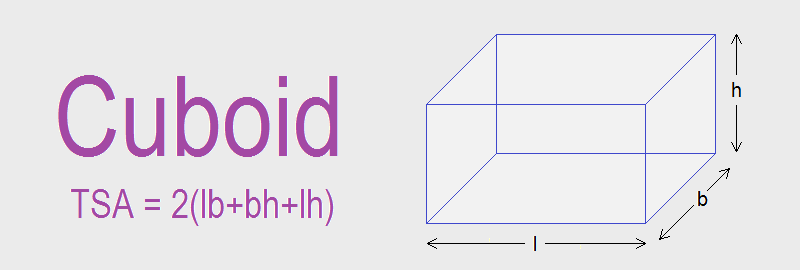# Cuboid## Cuboid

A cuboid is a solid object having six rectangular faces with opposite faces equal in shape and size. It is also a rectangular prism having same cross-section throughout its length or breadth or height.
The figure given above is a cuboid with six rectangular faces ABCD, EFGH, ABFE, DCGH, ADHE and BCGF where the opposite faces ABCD and EFGH (front and back), ABFE and DCGH (left and right), ADHE and BCGF (up and down) are equal in shape and size.

### Net of Cuboid

Net of cuboid is as given below:

### Total Surface Area of a Cuboid

The sum of the area of all rectangular faces of a cuboid is called the total surface area (TSA) of that cuboid. Let l, b and h be the length, breadth and height respectively of the cuboid ABCDEFGH given below.
Area of rectangular face BCGF = lb
Area of rectangular face ADHE = lb
Area of rectangular face DCGH = bh
Area of rectangular face ABFE = bh
Area of rectangular face ABCD = lh
Area of rectangular face EFGH = lh

Therefore, total surface area of cuboid = lb + lb + bh + bh + lh + lh
= 2lb + 2bh + 2lh
= 2(lb + bh + lh)

TSA of Cuboid = 2(lb + bh + lh)

### Workout Examples

Example 1: Find the total surface area of the given solid figure.
Solution: The given figure is a cuboid of,
Length (l) = 10cm
Height (h) = 5cm

TSA of cuboid = 2(lb + bh + lh)
= 2(10×6 + 6×5 + 10×5)
= 2(60 + 30 +50)
= 2 × 140
= 280cm2

Example 2: If the length, breadth and total surface area of a cuboid is 5m, 2m and 62m2 respectively, find its height.

Solution: Here,
Length (l) = 5m
Height (h) = ?
TSA of cuboid = 62m2
i.e.      2(lb + bh + lh) = 62
or,       2(5×2 + 2×h + 5×h) = 62
or,       2(10 + 2h + 5h) = 62
or,       2(10 + 7h) = 62
or,       20 + 14h = 62
or,       14h = 62 – 20
or,       14h = 42
or,       h = 42/14
or,       h = 3m

height of cuboid = 3m.

Example 3: The dimensions of a closed cuboidal packing case are in the ratio of 5:4:3. If the total surface area is 0.94m2, find the dimensions of the cuboid.

Solution: Here, length, breadth and height are in the ratio of 5:4:3. So let,
Length (l) = 5x
Height (h) = 3x
TSA of cuboid = 0.94m2
i.e.      2(lb + bh + lh) = 0.94
or,       2(5x×4x + 4x×3x + 5x×3x) = 0.94
or,       2(20x2 + 12x2 + 15x2) = 0.94
or,       2 × 47x2 = 0.94
or,       94x2 = 0.94
or,       x2 = 0.94/94
or,       x2 = 0.01
or,       x = 0.1m

Dimensions of cuboid are:
Length (l) = 5x = 5 × 0.1m = 0.5m
Breadth (b) = 4x = 4 × 0.1m = 0.4m
Height (h) = 3x = 3 × 0.1m = 0.3m

You can comment your questions or problems regarding the area and volume of cuboid here.